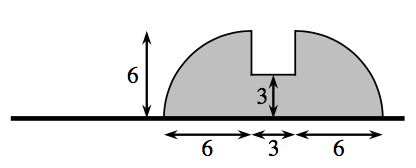### Home > APCALC > Chapter 6 > Lesson 6.1.3 > Problem6-37

6-37.

A flag is shown below.1. Imagine rotating the flag about its pole and describe the resulting three-dimensional figure. Draw a picture of this figure on your paper.

2 hemispheres with a cylinder between them.

2. Calculate the volume of the rotated flag.

$V=\frac{4}{3}\pi r^{3}_{\text{big}}+\pi r^{2}_{\text{small}}h$